# CHAPTER 4 PPT FORCE MOTION FORCE Force is

• Slides: 26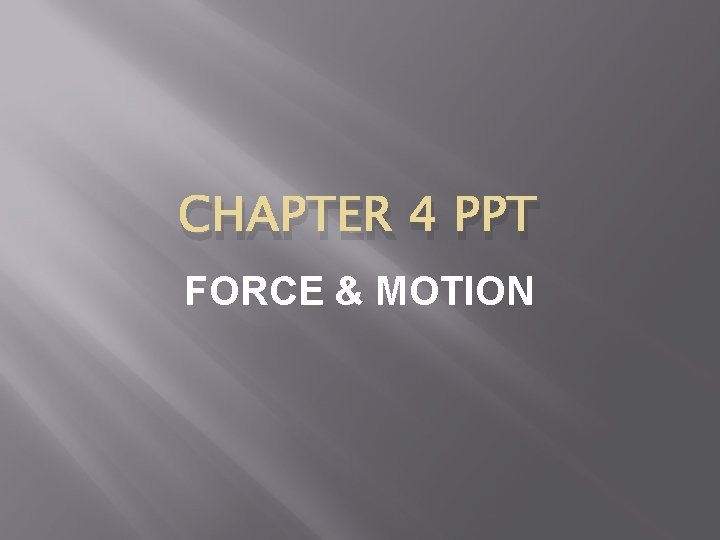CHAPTER 4 PPT FORCE & MOTIONFORCE Force is an agent which changes or tends to change the state of rest or of uniform motion of a body. Force can accelerate or decelerate a body. Force is a vector quantity. �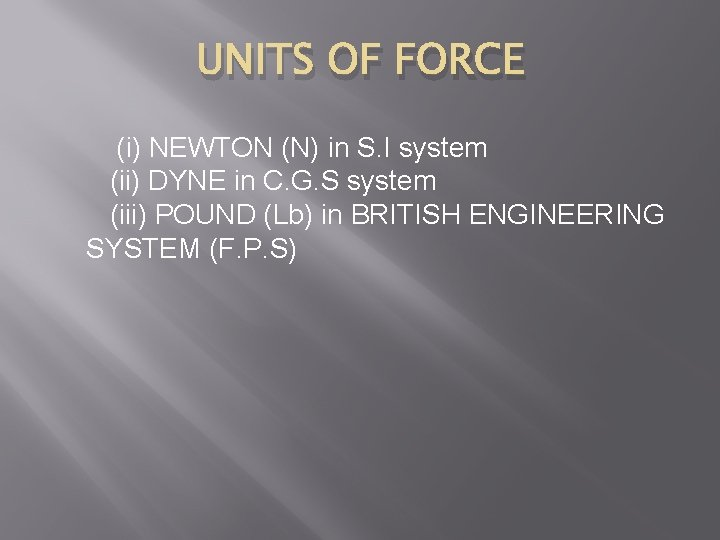UNITS OF FORCE (i) NEWTON (N) in S. I system (ii) DYNE in C. G. S system (iii) POUND (Lb) in BRITISH ENGINEERING SYSTEM (F. P. S)NEWTON � � � "The amount of force that produces an acceleration of 1 m/s 2 in a body of mass 1 -kg is equal to 1 NEWTON. " 1 N = 1 kg x 1 m/s 2 [ N = kg m/s 2]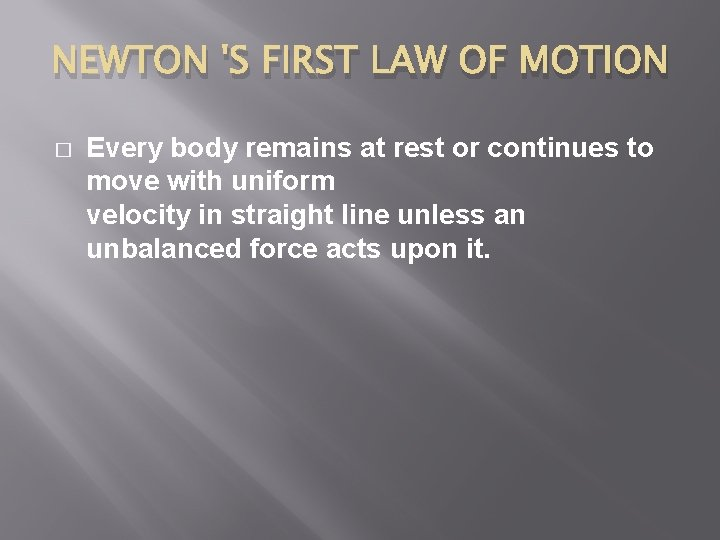NEWTON 'S FIRST LAW OF MOTION � Every body remains at rest or continues to move with uniform velocity in straight line unless an unbalanced force acts upon it.EXPLANATION � � � First law of motion consists of two parts: PART NO 1: The first part states that a body at rest remains at rest unless an unbalanced force acts upon it. This part is in accordance with our common experience for example, a book lying on a table remains at rest unless it is lifted or pushed by an external force. PART NO 2 : Second part states that a body in motion remains in motion with uniform velocity unless an unbalance force acts upon it. This part is not self-evident because a ball pushed once does not continue its motion forever. A little consideration however, shows that there is an opposing force like ground friction and air friction acting in this case. These frictional forces are responsible to stop the ball. If we eliminate these opposing forces, a body in motion will continue its motion forever.INERTIA � Tendency of a body by virtue of which the body at rest or moving with uniform velocity retains its state is called INERTIA.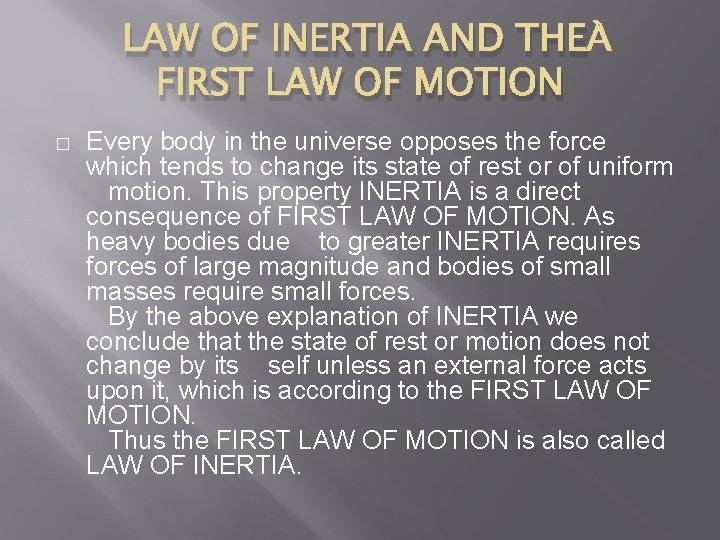LAW OF INERTIA AND THE FIRST LAW OF MOTION � Every body in the universe opposes the force which tends to change its state of rest or of uniform motion. This property INERTIA is a direct consequence of FIRST LAW OF MOTION. As heavy bodies due to greater INERTIA requires forces of large magnitude and bodies of small masses require small forces. By the above explanation of INERTIA we conclude that the state of rest or motion does not change by its self unless an external force acts upon it, which is according to the FIRST LAW OF MOTION. Thus the FIRST LAW OF MOTION is also called LAW OF INERTIA.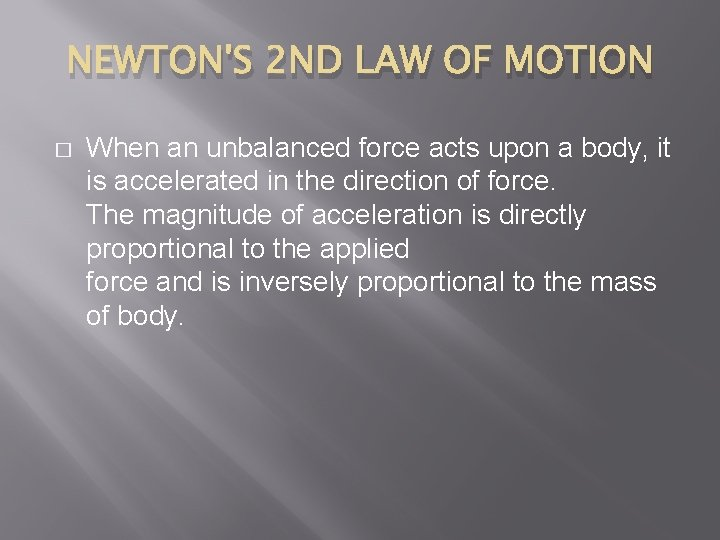NEWTON'S 2 ND LAW OF MOTION � When an unbalanced force acts upon a body, it is accelerated in the direction of force. The magnitude of acceleration is directly proportional to the applied force and is inversely proportional to the mass of body.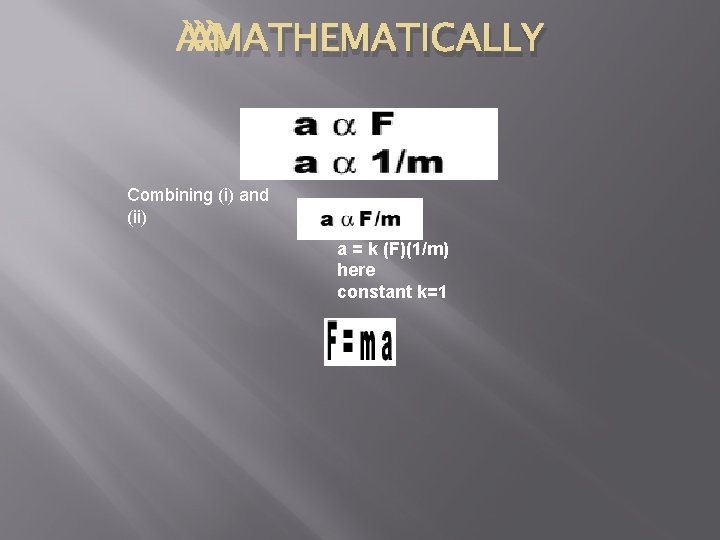MATHEMATICALLY Combining (i) and (ii) a = k (F)(1/m) here constant k=1NEWTON'S 3 RD LAW OF MOTION To every action there is a reaction equal in magnitude but opposite in direction. FACTION = -FREACTION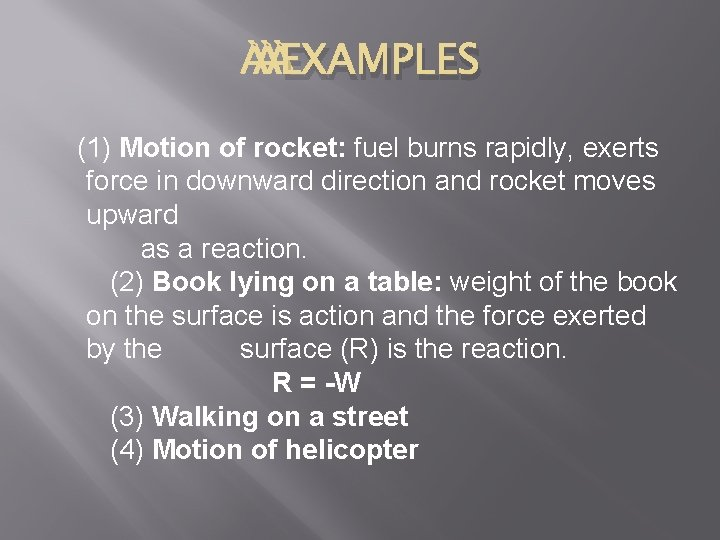EXAMPLES (1) Motion of rocket: fuel burns rapidly, exerts force in downward direction and rocket moves upward as a reaction. (2) Book lying on a table: weight of the book on the surface is action and the force exerted by the surface (R) is the reaction. R = -W (3) Walking on a street (4) Motion of helicopter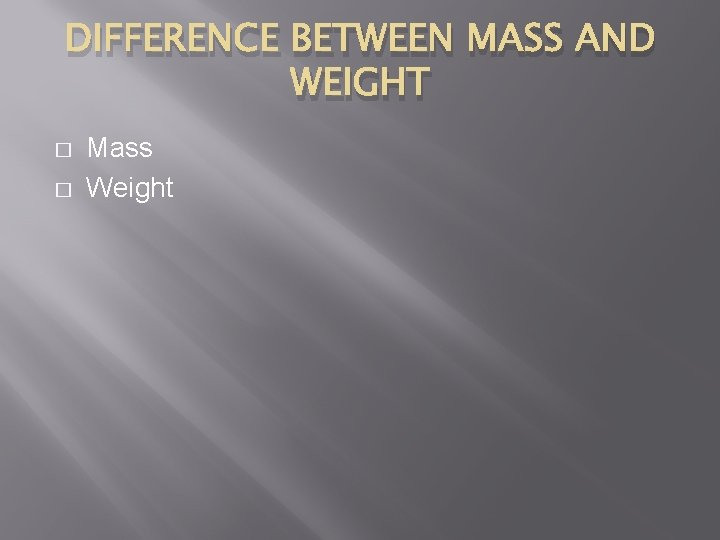DIFFERENCE BETWEEN MASS AND WEIGHT � � Mass WeightMass � � (1) The quantity of matter in a body is called its mass. (2) Mass is a scalar quantity. (3) Mass of a body is always constant every where in the universe. (4) Mass of a moving body is m=F/a.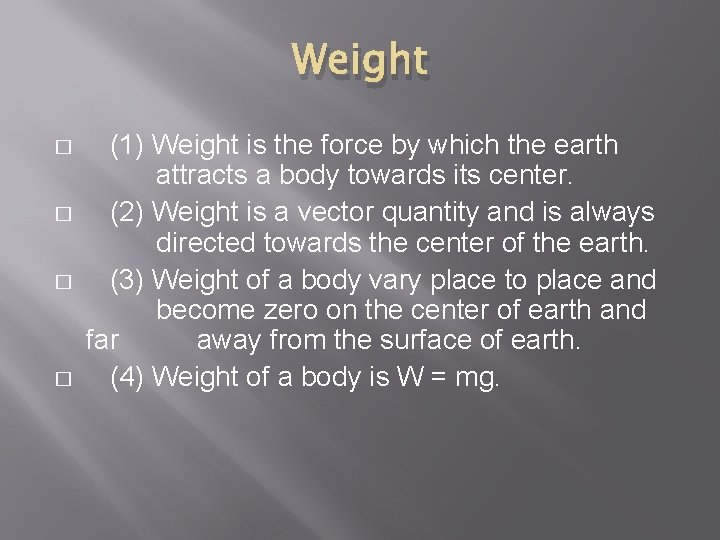Weight � � (1) Weight is the force by which the earth attracts a body towards its center. (2) Weight is a vector quantity and is always directed towards the center of the earth. (3) Weight of a body vary place to place and become zero on the center of earth and far away from the surface of earth. (4) Weight of a body is W = mg.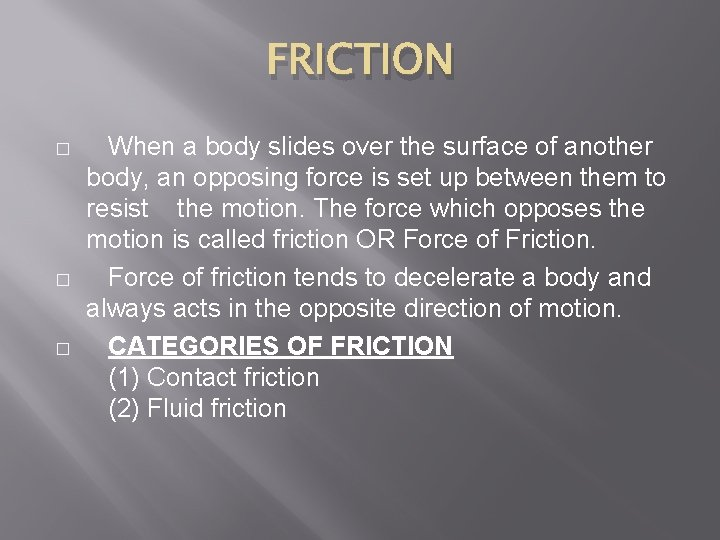FRICTION � � � When a body slides over the surface of another body, an opposing force is set up between them to resist the motion. The force which opposes the motion is called friction OR Force of Friction. Force of friction tends to decelerate a body and always acts in the opposite direction of motion. CATEGORIES OF FRICTION (1) Contact friction (2) Fluid friction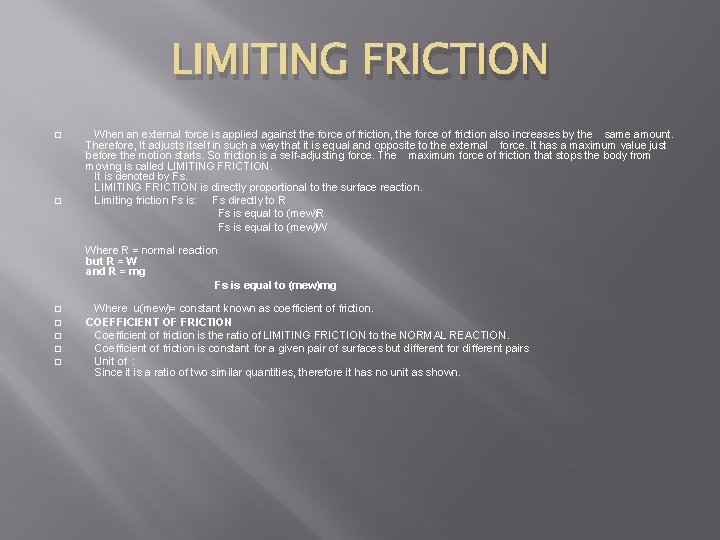LIMITING FRICTION When an external force is applied against the force of friction, the force of friction also increases by the same amount. Therefore, It adjusts itself in such a way that it is equal and opposite to the external force. It has a maximum value just before the motion starts. So friction is a self-adjusting force. The maximum force of friction that stops the body from moving is called LIMITING FRICTION. It is denoted by Fs. LIMITING FRICTION is directly proportional to the surface reaction. � Limiting friction Fs is: Fs directly to R Fs is equal to (mew)W � Where R = normal reaction but R = W and R = mg Fs is equal to (mew)mg � � � Where u(mew)= constant known as coefficient of friction. COEFFICIENT OF FRICTION Coefficient of friction is the ratio of LIMITING FRICTION to the NORMAL REACTION. Coefficient of friction is constant for a given pair of surfaces but different for different pairs Unit of : Since it is a ratio of two similar quantities, therefore it has no unit as shown.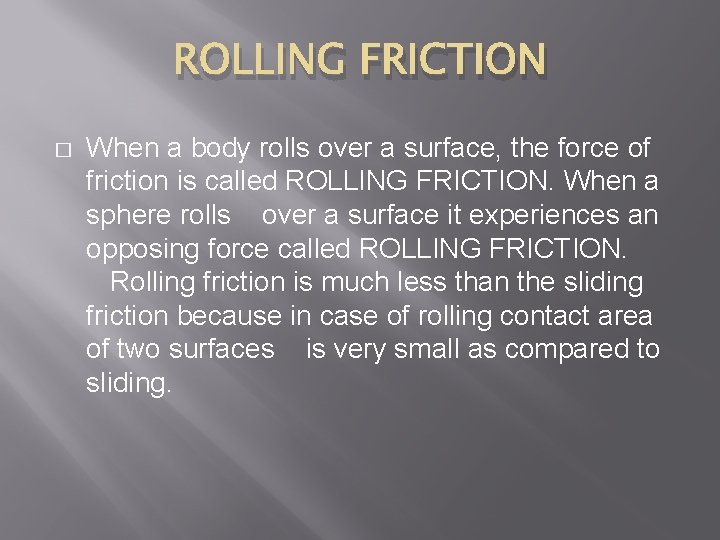ROLLING FRICTION � When a body rolls over a surface, the force of friction is called ROLLING FRICTION. When a sphere rolls over a surface it experiences an opposing force called ROLLING FRICTION. Rolling friction is much less than the sliding friction because in case of rolling contact area of two surfaces is very small as compared to sliding.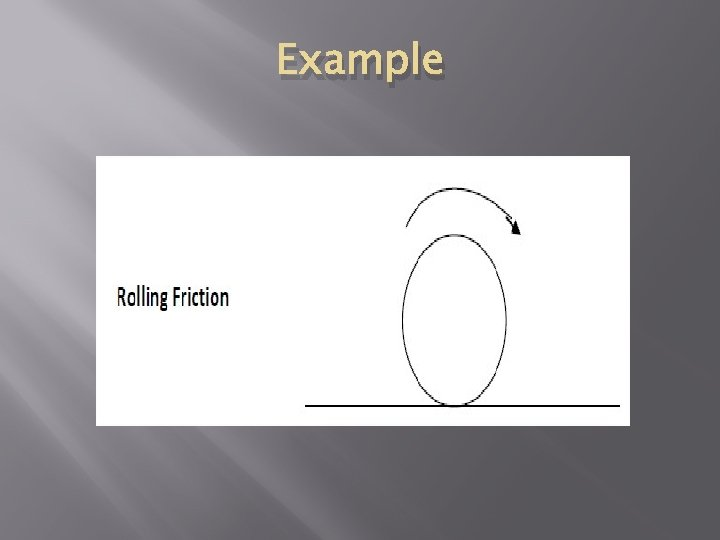ExampleMomentum- Law of conservation of Momentum MOMENTUM Quantity of motion of a body is referred to as "MOMENTUM". � Definition Momentum of a moving body defined as : � "the product of mass and velocity of a body is called MOMENTUM. " � Mathematically � Momentum = mass x velocity � It is a vector quantity. Momentum is always directed in the direction of velocity. The unit of momentum is in S. I system kg. m/s or NS. Momentum depends upon mass and velocity of body. �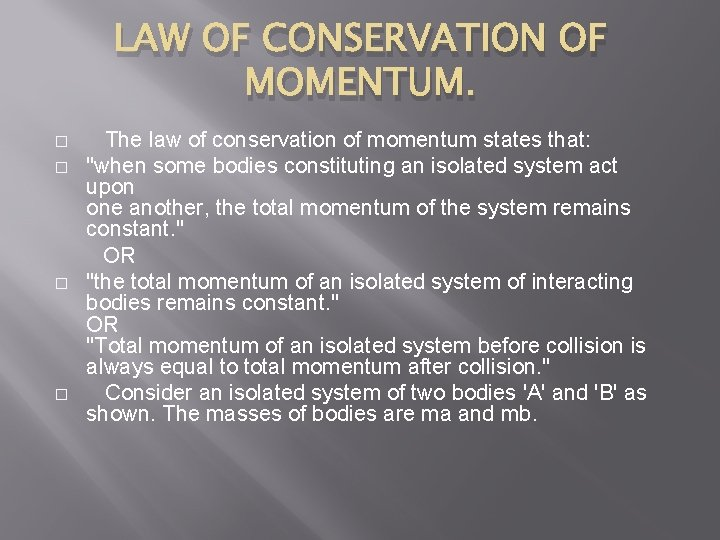LAW OF CONSERVATION OF MOMENTUM. The law of conservation of momentum states that: � "when some bodies constituting an isolated system act upon one another, the total momentum of the system remains constant. " OR � "the total momentum of an isolated system of interacting bodies remains constant. " OR "Total momentum of an isolated system before collision is always equal to total momentum after collision. " � Consider an isolated system of two bodies 'A' and 'B' as shown. The masses of bodies are ma and mb. �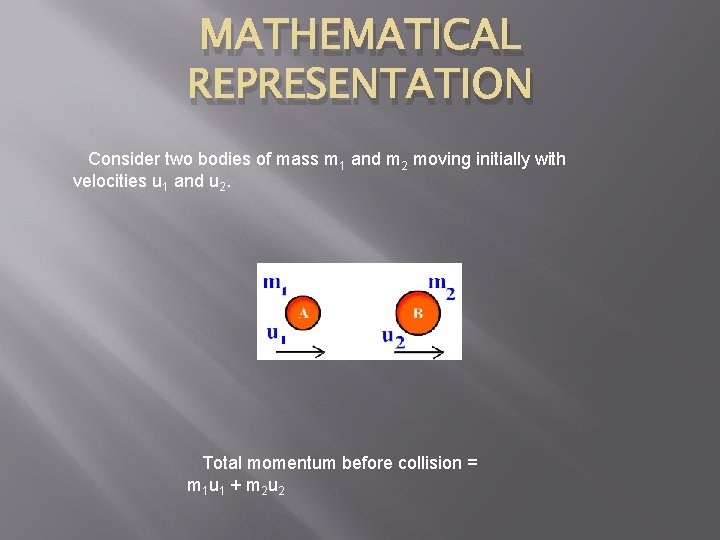MATHEMATICAL REPRESENTATION Consider two bodies of mass m 1 and m 2 moving initially with velocities u 1 and u 2. Total momentum before collision = m 1 u 1 + m 2 u 2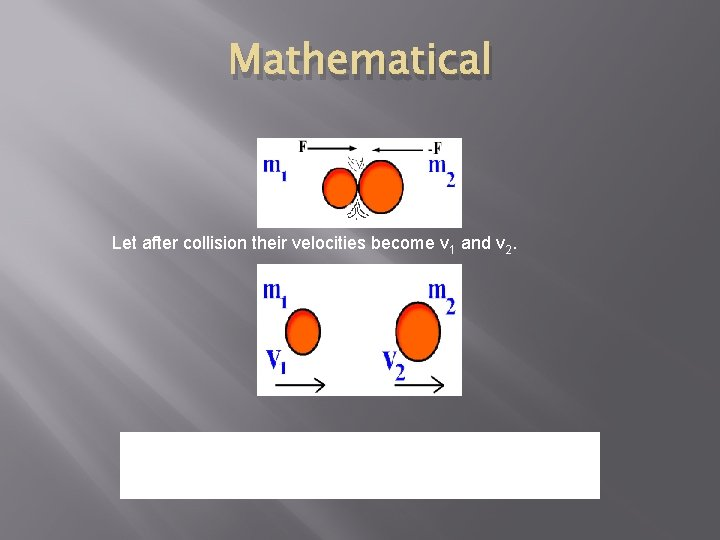Mathematical Let after collision their velocities become v 1 and v 2. Total momentum after collision = m 1 v 1 + m 2 v 2 According to the law of conservation of momentum m 1 u 1 + m 2 u 2 = m 1 v 1 + m 2 v 2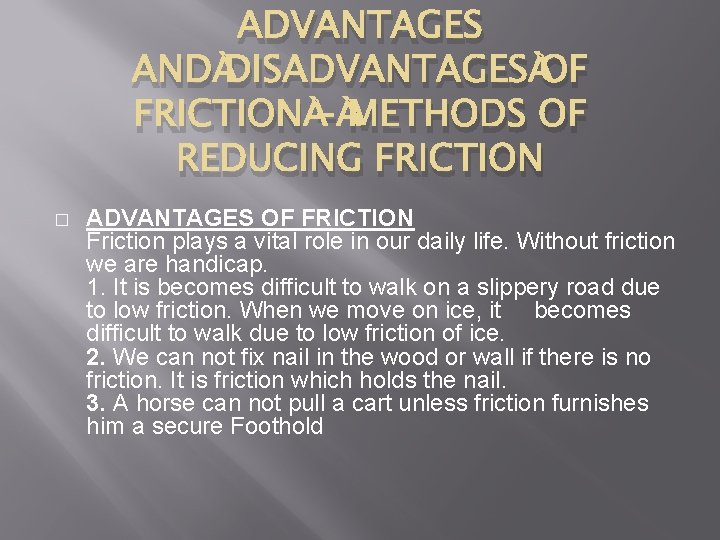ADVANTAGES AND DISADVANTAGES OF FRICTION - METHODS OF REDUCING FRICTION � ADVANTAGES OF FRICTION Friction plays a vital role in our daily life. Without friction we are handicap. 1. It is becomes difficult to walk on a slippery road due to low friction. When we move on ice, it becomes difficult to walk due to low friction of ice. 2. We can not fix nail in the wood or wall if there is no friction. It is friction which holds the nail. 3. A horse can not pull a cart unless friction furnishes him a secure Foothold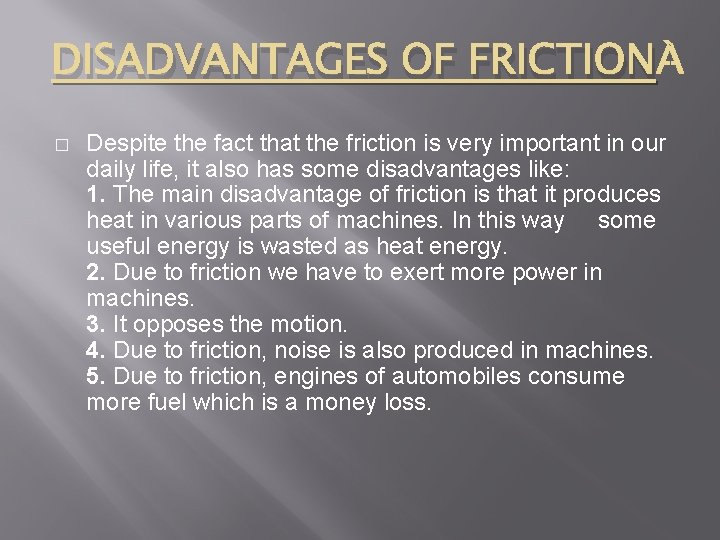DISADVANTAGES OF FRICTION � Despite the fact that the friction is very important in our daily life, it also has some disadvantages like: 1. The main disadvantage of friction is that it produces heat in various parts of machines. In this way some useful energy is wasted as heat energy. 2. Due to friction we have to exert more power in machines. 3. It opposes the motion. 4. Due to friction, noise is also produced in machines. 5. Due to friction, engines of automobiles consume more fuel which is a money loss.METHODS OF REDUCING FRICTION � There a number of methods to reduce friction in which some are discussed here. USE OF LUBRICANTS: The parts of machines which are moving over one another must be properly lubricated by using oils and lubricants of suitable viscosity. USE OF GREASE: Proper greasing between the sliding parts of machine reduces the friction. USE OF BALL BEARING: In machines where possible, sliding friction can be replaced by rolling friction by using ball bearings. DESIGN MODIFICATION: Friction can be reduced by changing the design of fast moving objects. The front of vehicles and airplanes made oblong to minimize friction.